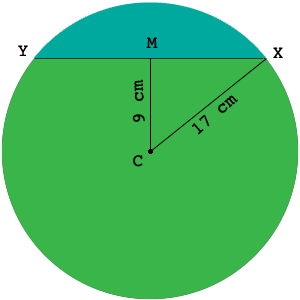SEARCH HOMEMath Central Quandaries & QueriesQuestion from Arwin: The radius of a circle is 17 cm. A chord XY lies 9 cm from the centre and divides the circle into two segments. Find the perimeter of the minor segment.Hi Arwin,In my diagram $M$ is the center of the circle. The minor segment is shaded blue and its perimeter is composed of two section, the chord and the arc of the circle from $X$ to $Y.$

The angle $XMC$ is a right angle (do you see why?) so Pythagoras Theorem allows you to find the distance from $X$ to $M.$ and hence the length of the chord $XY.$ The length of the arc from $X$ to $Y$ can be found using the information in Harley's response to an earlier question. Make sure you have you calculator set to radians rather than degrees.

PennyMath Central is supported by the University of Regina and The Pacific Institute for the Mathematical Sciences.